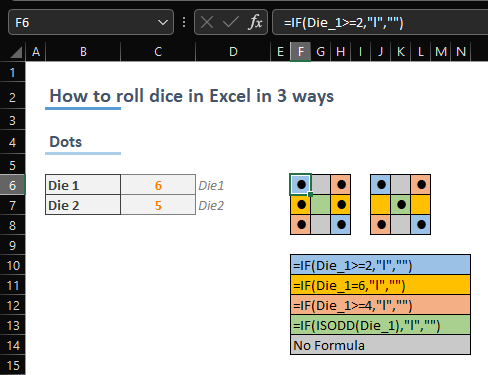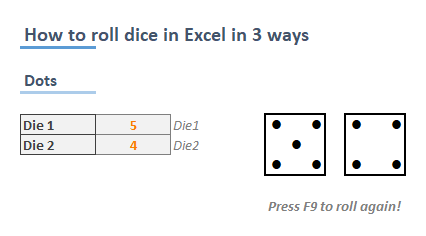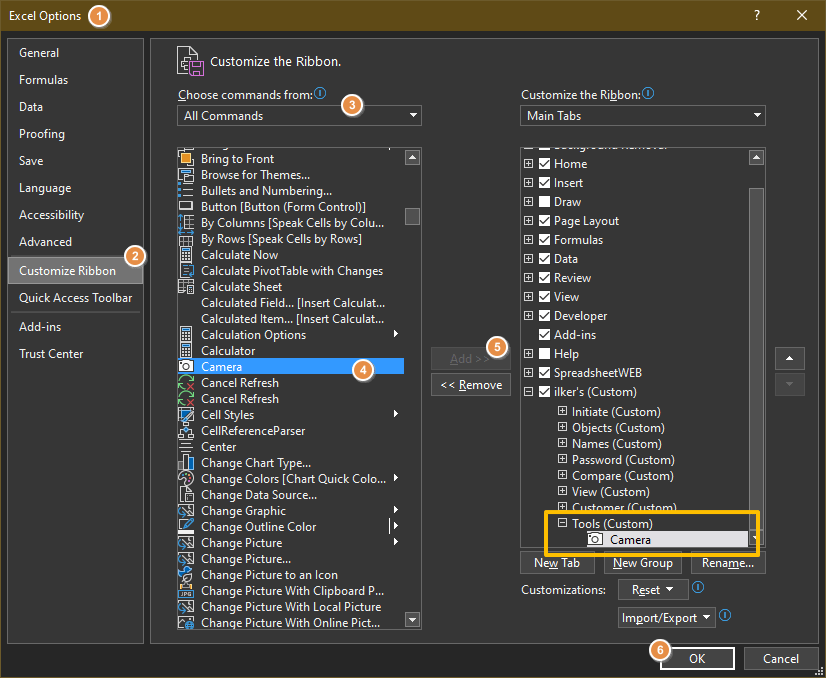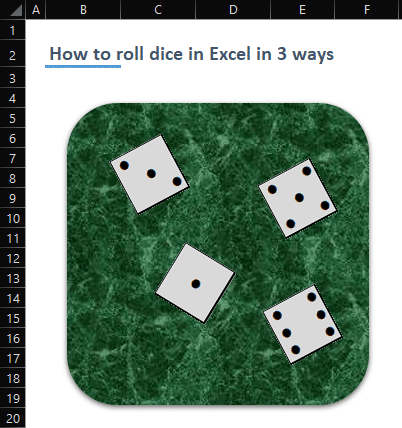The throw of dice is a common way of generating random numbers. It's not necessary to talk about the role of dice in board and role-playing games. Although, a simple formula can give what you need easily, you can make the dice rolling fancy in Excel. In this guide, we’re going to show you How to roll dice in Excel in 3 ways.

## Formula Way

Excel has three formulas for generating random numbers:

• RAND: Returns a random decimal number between 0 and 1.
• RANDBETWEEN: Returns an integer between specified upper and lower limits.
• RANDARRAY: Returns one or multiple decimal or integer numbers between specified upper and lower limits.

Obviously, a dice consists of six sides, representing six integers from 1 to 6. Thus, using integer-returning functions RANDBETWEEN and RANDARRAY suit our cause. Also, you can use the newer RANDARRAY function that was released to replace both legacy RAND and RANDARRAY functions. However, Microsoft allows this function and dynamic array features for the Microsoft 365 subscribers.

Every time the workbook is calculated, each function returns a random generated number. You can do the calculation by pressing the F9 key on your keyboard. To make it fancy, you can create a button which triggers the calculation. All you need to do is to record a macro, press the F9 and stop recording. Now, you can assign your macro into a command button.

### RANDBETWEEN

All you need to do is to give lower and upper limits to the function. You need to copy and paste the formula if you need to roll multiple dice.

=RANDBETWEEN(1,6)### RANDARRAY

Due to its flexibility, RANDARRAY function is not as direct as the RANDBETWEEN. However, the ability to fill multiple cells with a single formula is a killer feature.

The RANDARRAY function needs number of rows and columns to be filled; minimum and maximum values, and a Boolean value to determine to return integer or decimal values.

For example, our sample formula fills 3 rows by 2 column range with integer numbers between 1 and 6:

=RANDARRAY(3,2,1,6,TRUE)A single formula is enough to fill a 3 * 2 range. Press the F9 key to see different rolls.

Although the formulas can give the numbers we need, they are just numbers. Boring! Let's see what we can do to get a cooler look.

## Rolling Dice in Excel with Dots

You might have already thought using cells to generate a dice shape. But creating dots in a dice might seem like a problem at first. Thanks to the Wingdings font support of Excel, you can display big dots easily. Let's start.

We will use two dice formulas to keep things easy. Each dice will need its own cell. Since dots on a dice are separated in 9 cells (3*3) , we will need a similar range of cells with equal row heights and column widths.Once the dice boxes are ready, select them and set Wingdings as their font. After that, type "l" (lowercase L without quotes) into boxes to see if the dot looks as you want. You may want to change the font size.Now, it's time to add formulas but a reminder first: We named our cells as Dice_1 (C6) and Dice_2 (C7) to ease the formula writing.

The formulas will simply display or hide the dots according to the dice value. Thus, the logic should be consistent with the dice layout. For example, the center dot is available for only 1, 3 and 5. On the other hand, the dot at top left and bottom right, is not available for 1 only.

Here are the formulas:

 2, 3, 4, 5, 6 =IF(Die_1>=2,"l","") 6 =IF(Die_1=6,"l","") 4, 5, 6 =IF(Die_1>=4,"l","") 1, 3, 5 =IF(ISODD(Die_1),"l","")The ISODD function returns TRUE if the number argument is an odd number, or FALSE if it's an even number. It allows a shorter formula rather than multiple ifs or conditions.

You can also remove the coloring and borders to obtain more real-life dice look.## Camera Tool for Even More

Our final approach is a hidden feature of Excel: Camera Tool. Excel has a tool that allows you to take a dynamic snapshot of a range as an image. Once the image is created, you are not bound to cell height and width and font sizes. You can adjust its dimensions or rotation as you want.

1. Open Excel Options under File.After created, you can move or modify your image.